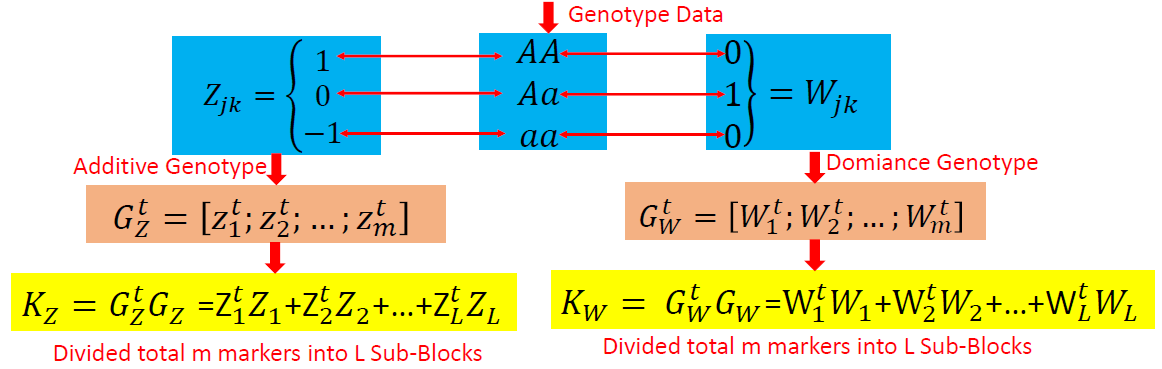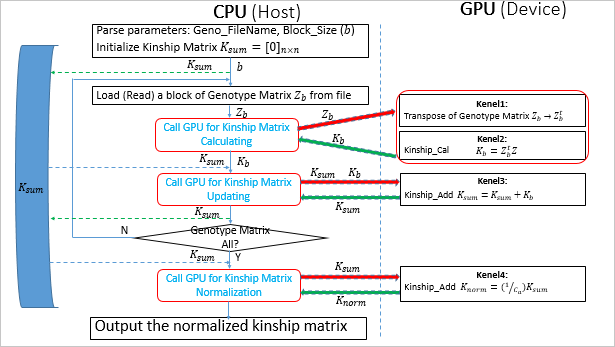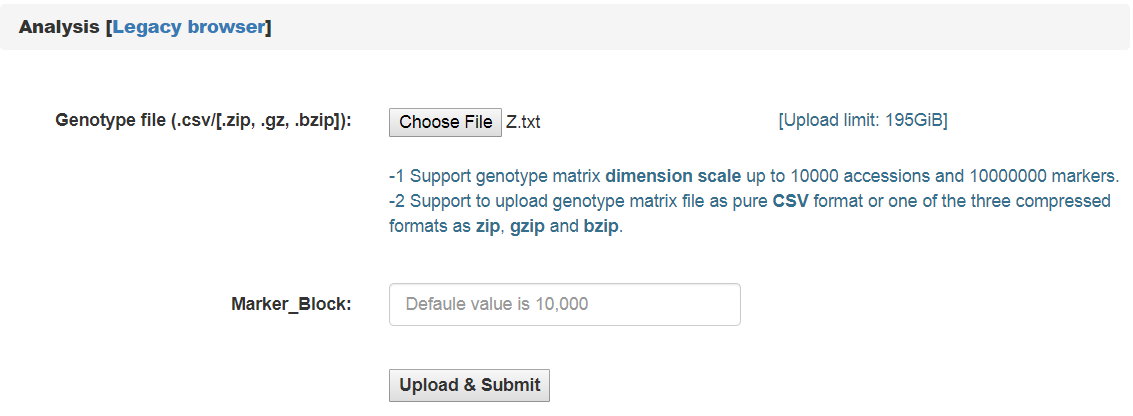#### Overview

Recently, high-throughput sequencing, particularly NGS technology make it capable to sequence and discover great huge number of SNPs and furtherly explore the within-species diversity via constructing haplotype maps and conducting genome-wide association studies(GWAS). To conduct GWASs, one type of (Linear Mixed Models)LMMs is always applied and the calculating of the kinship matrix is usually the first step to solve the LMMs. The kinship matrix K is a 2D matrix with the dimension of nxn, and each kinship matrix entry K(i,j) is a coefficient to assess the genetic resemblance between individual i and individual j. In principle, the larger the individual number, and the larger the genotypic marker number, the more complexity the kinship matrix calculating. A typical GWAS studies may call several millions scale SNPs and the genotypic markers across several hundred ~ thousands accessions/lines. Therefore, a typical genotypic data can amount to >100 GBs. To load such a GBs scale genotypic data and implement the kinship matrix calculation is a challenge

In the recent years, GPU (Graphics Processing Units) with multiple hardware processor (>1,000) cores has become a standard HPC (High Performance Computing) solution system for large scale computing. Especially, GPU empowered HPC platform favor to large scale matrix operation.

We have analyzed the math principle and the complexity of the marker-assist kinship matrix, then we have successfully developed this GPU empowered pipeline for main effect kinship matrix calculating: KMC1D , which can easily realize several hundreds of times of speeding up, when compared with the golden single processing.

#### Rationale

In genetic analysis, there are two kinds of main effects: additive and dominant. If the genotype data are available, the additive and dominant genotypic numeric values can be clearly defined. To calculate the kinship matrix, the simplest method is to load the whole the genotype matrix data for its transpose and then the multiplication of two matrix, which in principle need to request the CPU to allocate a memory at the same size of the genotype data file. Obviously, it's clumsy and even possible, if the original genotype data amount to 100GBs . In mathematically principle, the kinship matrix is of linearity, which can be acquired by summing a bunch of sub-kinship matrix, and each sub-kinship matrix is calculated by a block of successive markers. Therefore, if we only load one block of the genotypic markers, such as 10000, which will greatly reduce the memory requirements. Fig.1 depict the math rationale to generate the two types of genotypic marker values and partition all the markers into successive blocks for sub kinship matrix calculating.Fig. 1 The math rationale to generate the two types of main genotypic values and partition all the markers into successive blocks for sub kinship matrix calculating

GPU based parallel computing favors to compute large scale matrix operations. Ideally, each matrix entry operation can be implemented by one thread corresponds to one GPU core. All the GPU program include two parts: one as the host part running on CPU, and the other part as kernel codes running on the slavery device-GPU cores. The GPU kernel codes are functionally implemented by parallelization and distinguished by specific primitive _global_. We analyzed the mathematical principle and the matrix operation procedure to calculate kinship matrix, and coded 4 GPU core paralleling kernel functions including transpose of matrix, multiplication of two matrix, sum of two matrix, and the normalization of the summed kinship matrix. Fig.2 depict the GPU empowered parallel pipeline architecture for main effect kinship matrix calculating through partitioning coded genotypic marker into successive blocks, then calculating sub kinship matrix and merging into a whole.Fig. 2 GPU empowered parallel pipeline architecture for main effect kinship matrix calculating

To efficiently load the huge genotypic data, we developed a module which can work in HTML5 browser for a resumable multithreading chunked data uploading.

#### Representative Application of KMC1D

Equipped with GPU parallel computing, KMC1D can calculate the main effect kinship matrix for the given coded genotype data. It need either the additive effect matrix (Z_matrix) or the dominance effect matrix(W_matrix) file. Fig.3 provide the user-interface snapshot for additive effect kinship matrix calculating. Note: The inputting genotypic matrix file must be comma or Tab delimited text file, and stored as m(rows, Markers) x n(cols, Individual/Accessions), each row corresponds to one marker .Fig. 3 The user-interface for additive effect kinship matrix calculating

#### References

1. Xu, S., "Mapping Quantitative Trait Loci by Controlling Polygenic Background Effects". Genetics, 2013. 195(4):p.1709-23.

2. Zhang W., Dai X., Wang Q., Xu S., Zhao P.X., "PEPIS: A Pipeline for Estimating Epistatic Effects in Quantitative Trait Locus Mapping and Genome-Wide Association Studies", 2016. PLoS Comput Biol, 12(5)

3. Cecilia J. M. , Garc´ıa J. M. , and Ujaldon M., “The GPU on the Matrix-Matrix Multiply: Performance Study and Contributions”, in Parallel Computing: From Multicores and GPU’s to Petascale, B. Chapman et al., Eds. Advances in Parallel Computing, vol. 19, pp. 331-340, 2010.

4. Dobravec T., Bulic P., "Comparing CPU and GPU Implementations of a Simple Matrix Multiplication Algorithm", IJCEE, vol 9, 430-438, 2017.